# US8160846B2 - Method of modeling phase changes due to laser pulse heating - Google Patents

Method of modeling phase changes due to laser pulse heating Download PDF

## Info

Publication number
US8160846B2
US8160846B2 US12/453,656 US45365609A US8160846B2 US 8160846 B2 US8160846 B2 US 8160846B2 US 45365609 A US45365609 A US 45365609A US 8160846 B2 US8160846 B2 US 8160846B2
Authority
US
United States
Prior art keywords
δ
represents
substrate material
liquid
ρ
Prior art date
Legal status (The legal status is an assumption and is not a legal conclusion. Google has not performed a legal analysis and makes no representation as to the accuracy of the status listed.)
Active, expires
Application number
US12/453,656
Other versions
US20100292965A1 (en
Inventor
Bekir Sami Yilbas
Current Assignee (The listed assignees may be inaccurate. Google has not performed a legal analysis and makes no representation or warranty as to the accuracy of the list.)
King Fahd University of Petroleum and Minerals
Original Assignee
King Fahd University of Petroleum and Minerals
Priority date (The priority date is an assumption and is not a legal conclusion. Google has not performed a legal analysis and makes no representation as to the accuracy of the date listed.)
Filing date
Publication date
Application filed by King Fahd University of Petroleum and Minerals filed Critical King Fahd University of Petroleum and Minerals
Priority to US12/453,656 priority Critical patent/US8160846B2/en
Assigned to KING FAHD UNIV. OF PETROLEUM & MINERALS reassignment KING FAHD UNIV. OF PETROLEUM & MINERALS ASSIGNMENT OF ASSIGNORS INTEREST (SEE DOCUMENT FOR DETAILS). Assignors: MANSOUR, SAAD BIN, YILBAS, BEKIR SAMI
Publication of US20100292965A1 publication Critical patent/US20100292965A1/en
Application granted granted Critical
Publication of US8160846B2 publication Critical patent/US8160846B2/en
Application status is Active legal-status Critical

• 238000004089 heat treatment Methods 0 abstract claims description title 51
• 239000000758 substrates Substances 0 abstract claims description 88
• 239000000463 materials Substances 0 abstract claims description 79
• 239000011797 cavity materials Substances 0 abstract claims description 53
• 238000004093 laser heating Methods 0 abstract claims description 22
• 239000007788 liquids Substances 0 claims description 58
• 239000007787 solids Substances 0 claims description 40
• 239000007790 solid phases Substances 0 claims description 21
• 238000002844 melting Methods 0 claims description 19
• 238000004364 calculation methods Methods 0 claims description 18
• 239000007791 liquid phases Substances 0 claims description 16
• 239000000727 fractions Substances 0 claims description 9
• 238000009835 boiling Methods 0 claims description 7
• 230000001419 dependent Effects 0 abstract description 4
• 238000002310 reflectometry Methods 0 claims description 3
• 230000001965 increased Effects 0 description 24
• 238000001704 evaporation Methods 0 description 17
• 238000000034 methods Methods 0 description 13
• 238000009826 distribution Methods 0 description 9
• 230000002123 temporal effects Effects 0 description 7
• 230000015572 biosynthetic process Effects 0 description 5
• 238000001816 cooling Methods 0 description 5
• 238000005755 formation Methods 0 description 5
• 238000004088 simulation Methods 0 description 5
• 230000000875 corresponding Effects 0 description 4
• 230000001603 reducing Effects 0 description 4
• 230000003993 interaction Effects 0 description 3
• 239000010410 layers Substances 0 description 3
• 229910052757 nitrogen Inorganic materials 0 description 3
• 230000036961 partial Effects 0 description 3
• 229910052717 sulfur Inorganic materials 0 description 3
• 229910001868 water Inorganic materials 0 description 3
• 229910000831 Steel Inorganic materials 0 description 2
• 229910052775 Thulium Inorganic materials 0 description 2
• 230000000694 effects Effects 0 description 2
• 239000007789 gases Substances 0 description 2
• 229910052739 hydrogen Inorganic materials 0 description 2
• 230000016507 interphase Effects 0 description 2
• 230000015654 memory Effects 0 description 2
• 239000000203 mixtures Substances 0 description 2
• 239000010959 steel Substances 0 description 2
• 229910052721 tungsten Inorganic materials 0 description 2
• 238000004458 analytical methods Methods 0 description 1
• 229910002056 binary alloys Inorganic materials 0 description 1
• 229910052799 carbon Inorganic materials 0 description 1
• 230000003247 decreasing Effects 0 description 1
• 230000004069 differentiation Effects 0 description 1
• 229910052731 fluorine Inorganic materials 0 description 1
• 239000011133 lead Substances 0 description 1
• 239000010912 leaf Substances 0 description 1
• 239000011344 liquid materials Substances 0 description 1
• 239000002184 metal Substances 0 description 1
• 229910052751 metals Inorganic materials 0 description 1
• 238000006011 modification Methods 0 description 1
• 230000004048 modification Effects 0 description 1
• 230000021715 photosynthesis, light harvesting Effects 0 description 1
• 230000000704 physical effects Effects 0 description 1
• 230000002250 progressing Effects 0 description 1
• 230000004044 response Effects 0 description 1
• 230000036633 rest Effects 0 description 1
• 230000001340 slower Effects 0 description 1
• 239000011343 solid materials Substances 0 description 1
• 230000001629 suppression Effects 0 description 1
• 230000001131 transforming Effects 0 description 1

## Images

•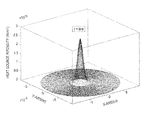•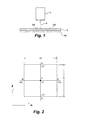•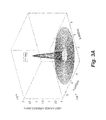•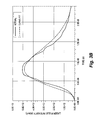•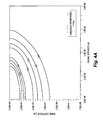•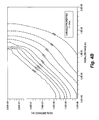•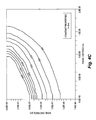•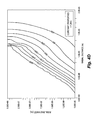••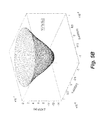•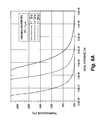•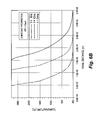•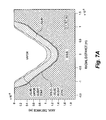•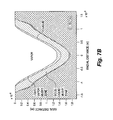•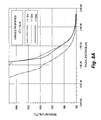•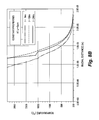•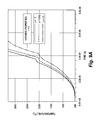•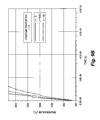•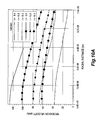•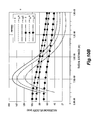•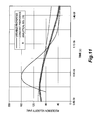•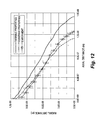•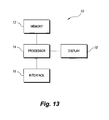## Classifications

• G06F30/20
• G06F2119/08

## Abstract

The method of modeling phase changes due to laser pulse heating utilizes energy equations and a discretizing numerical method to model temperature variation and cavity depth in a substrate material due to laser heating. Both constant and temperature-dependent thermal properties cases are considered.

## Description

BACKGROUND OF THE INVENTION

1. Field of the Invention

The present invention relates to numerical modeling and simulation methods, and particularly to a method of modeling phase changes due to laser pulse heating that utilizes energy equations and a discretizing numerical method to model temperature variation and cavity depth in a substrate material due to laser heating.

2. Description of the Related Art

In thermodynamics, a phase transition is the transformation of a thermodynamic system from one phase to another. At phase-transition point, physical properties may undergo abrupt changes, such as changes in volume, for example. Phase transitions, such as those caused by laser heating of a substrate material, typically occur between solid and liquid phases, and between liquid and vapor phases.

First-order phase transitions are those that involve a latent heat. During such a transition, a system either absorbs or releases a fixed (and typically large) amount of energy. During this process, the temperature of the system will stay constant as heat is added. Because energy cannot be instantaneously transferred between the system and its environment, first-order transitions are associated with “mixed-phase regimes” in which some parts of the system have completed the transition and others have not. This phenomenon is familiar to anyone who has boiled a pot of water, i.e., the water does not instantly turn into gas, but forms a turbulent mixture of water and water vapor bubbles. Mixed-phase systems are difficult to study, because their dynamics are violent and hard to control. However, many important phase transitions fall in this category, including the solid/liquid/gas transitions.

The equations governing such phase transitions are complex and, in some cases, impossible to solve analytically. Numeric methods must be applied, and since numerical techniques for partial differential equations often require a large amounts of time and computing power, it is difficult to develop effective numerical techniques for the calculation of phase-change related properties.

Thus, a method of modeling phase changes due to laser pulse heating solving the aforementioned problems is desired.

SUMMARY OF THE INVENTION

The method of modeling phase changes due to laser pulse heating utilizes energy equations and a discretizing numerical method to model temperature variation and cavity depth in a substrate material due to laser heating. Both constant and temperature-dependent thermal properties cases are considered. The method includes the steps of:

• (a) establishing a set of variables S0, Io, δ, rf, a, t, r, T, z, ρs, cps and ks, wherein the variable S0 represents a volumetric source term, the variable Io represents a laser peak power intensity, the variable δ represents an absorption coefficient for a substrate material, the variable rf represents reflectivity of the substrate material, the variable a represents a Gaussian parameter, the variable t represents time, the variable r represents distance measured along a radial direction, T represents temperature, z represents an axial distance, ρs represents a solid density, cps represents a specific heat capacity of a solid phase of the substrate material, and ks represents a thermal conductivity of the solid phase of the substrate material;
• (b) calculating temperature variation and cavity depth in the substrate material due to laser heating for solid and liquid heating of the substrate material as

$∂ ∂ t ⁢ ( ρ s ⁢ c p s ⁢ T ) = 1 r ⁢ ∂ ∂ r ⁢ ( k s ⁢ r ⁢ ∂ T ∂ r ) + ∂ ∂ z ⁢ ( k s ⁢ ∂ T ∂ z ) + S o ,$
wherein

$S o = I o ⁢ δ ⁡ ( 1 - r f ) ⁢ exp ⁡ ( - δ ⁢ ⁢ z ) ⁢ exp ( - r 2 a 2 ) ;$

• (c) establishing boundary conditions for the calculation of temperature variation and cavity depth in the substrate material for solid and liquid heating;
• (d) establishing a set of variables ρm, Lm, xm, and km, wherein ρm represents a density at a solid-liquid interface, Lm represents a latent heat of melting, xm represents a mass fraction of liquid, and km represents a thermal conductivity at the solid-liquid interface of the substrate material;
• (e) calculating temperature variation and cavity depth in the substrate material due to laser heating at the solid-liquid interface as

$ρ m ⁢ L m ⁢ ∂ x m ∂ t = 1 r ⁢ ∂ ∂ r ⁢ ( k m ⁢ r ⁢ ∂ T ∂ r ) + ∂ ∂ z ⁢ ( k m ⁢ ∂ T ∂ z ) + S o ;$

• (f) establishing a set of variables ρb, Lb, xb, and kb, wherein ρb represents a density at a liquid-vapor interface, Lb represents a latent heat of boiling, xb represents a mass fraction of vapor, and kb represents a thermal conductivity at the liquid-vapor interface of the substrate material;
• (g) calculating temperature variation and cavity depth in the substrate material due to laser heating at the liquid-vapor interface as

$ρ b ⁢ L b ⁢ ∂ x b ∂ t = ∂ ∂ r ⁢ ( k b ⁢ r ⁢ ∂ T ∂ r ) + ∂ ∂ z ⁢ ( k b ⁢ ∂ T ∂ z ) + S o ,$
wherein calculation of temperature variation and cavity depth in the substrate material due to laser heating for solid and liquid heating, at the solid-liquid interface, and at the liquid-vapor interface is performed by discretization; and

• (h) displaying numerical results of the calculated temperature variation and cavity depth in the substrate material due to laser heating for solid and liquid heating at the solid-liquid interface and at the liquid-vapor interface.

In the above, the boundary conditions for the calculation of temperature variation and cavity depth in the substrate material at the solid-liquid interface are set as T for both r and z are modeled as being an infinite distance from the substrate material being equal to T0, wherein T0 represents an initial temperature of the substrate material. Further, at an axis of symmetry of the substrate material,

$∂ T ⁡ ( 0 , z , t ) ∂ r - 0 ,$
and at a surface of the substrate material,

$k ⁢ 0 ⁢ T ⁢ ( T , 0 , t ) ∂ z = h ⁡ ( T ⁡ ( r , 0 , t ) - T 0 ) ,$
wherein h represents a convective heat transfer coefficient at the surface of the substrate material and k represents thermal conductivity at the surface of the substrate material. An energy content ΔU of a differential element having a volume Δ∀ at a melting temperature Tm of the substrate material in the calculation of temperature variation and cavity depth at the solid-liquid interface is given by ΔU=ρmΔ∀└xm(Lm+cp m (Tm−Tref))+cp s (1−xm)(Tm−Tref)┘, wherein cpm represents a specific heat capacity at the solid-liquid interface and Tref represents a reference temperature. The mass fraction of liquid xm is given by

$x m = m m m m + m s ,$
wherein mm represents a liquid mass and ms represents a solid mass.

The discretization is performed using the following steps:

• (a) establishing a set of variables ρ, p, i, j, a, b, C, E, W, N, S and H, wherein p represents a time index, i represents an r-coordinate index, j represents a z-coordinate index, ρ represents density, and a, b, C, F, W, N, S and H are intermediate variables;
• (b) discretizing the calculation of temperature variation and cavity depth in the substrate material due to laser heating for solid and liquid heating as

$a C ⁢ T i , j p = a E ⁢ T i + 1 , j p + a W ⁢ T i - 1 , j p + a N ⁢ T i , j + 1 p + a S ⁢ T i , j - 1 p + T i , j p - 1 + a H ⁢ S i , j p , ⁢ wherein$ $a E = αΔ ⁢ ⁢ t ⁡ ( r i + 0.5 ⁢ Δ ⁢ ⁢ r ) r i ⁡ ( Δ ⁢ ⁢ r ) 2 , a W = αΔ ⁢ ⁢ t ⁡ ( r i - 0.5 ⁢ Δ ⁢ ⁢ r ) r i ⁡ ( Δ ⁢ ⁢ r ) 2 , ⁢ a N = a S = αΔ ⁢ ⁢ t ( Δ ⁢ ⁢ z ) 2 , a H = Δ ⁢ ⁢ t ρ ⁢ ⁢ Cp , and ⁢ ⁢ a C = 1 + a E + a W + a N + a S ;$

• (c) discretizing the calculation of temperature variation and cavity depth in the substrate material due to laser heating for the solid-liquid interface as:

$x bi , j p = c E ⁢ T i + 1 , j p - c C ⁢ T i , j p + c W ⁢ T i - 1 , j p + c Z ⁡ ( T i , j + 1 p - 2 ⁢ T i , j p + T i , j - 1 p ) + c H ⁢ S i , j p + x bi , j p - 1 , ⁢ ⁢ wherein$ $⁢ c E = α b ⁢ Cp b ⁢ Δ ⁢ ⁢ t ⁡ ( r i + 0.5 ⁢ Δ ⁢ ⁢ r ) L b ⁢ r i ⁡ ( Δ ⁢ ⁢ r ) 2 , ⁢ c W = α b ⁢ Cp b ⁢ Δ ⁢ ⁢ t ⁡ ( r i - 0.5 ⁢ Δ ⁢ ⁢ r ) L b ⁢ r i ⁡ ( Δ ⁢ ⁢ r ) 2 , ⁢ ⁢ c C = c E + c W , c N = c S = α b ⁢ Cp b ⁢ Δ ⁢ ⁢ t L b ⁡ ( Δ ⁢ ⁢ z ) 2 , and ⁢ ⁢ b H = Δ ⁢ ⁢ t ρ b ⁢ L b ;$

• (d) discretizing the calculation of temperature variation and cavity depth in the substrate material due to laser heating for the liquid-vapor interface as:

$x bi , j p = c E ⁢ T i + 1 , j p - c C ⁢ T i , j p + c W ⁢ T i - 1 , j p + c Z ⁡ ( T i , j + 1 p - 2 ⁢ T i , j p + T i , j - 1 p ) + c H ⁢ S i , j p + x bi , j p - 1 , ⁢ ⁢ wherein$ $⁢ c E = α b ⁢ Cp b ⁢ Δ ⁢ ⁢ t ⁡ ( r i + 0.5 ⁢ Δ ⁢ ⁢ r ) L b ⁢ r i ⁡ ( Δ ⁢ ⁢ r ) 2 , c W = α b ⁢ Cp b ⁢ Δ ⁢ ⁢ t ⁡ ( r i - 0.5 ⁢ Δ ⁢ ⁢ r ) L b ⁢ r i ⁡ ( Δ ⁢ ⁢ r ) 2 , ⁢ ⁢ c C = c E + c W , ⁢ c N = c S = α b ⁢ Cp b ⁢ Δ ⁢ ⁢ t L b ⁡ ( Δ ⁢ ⁢ z ) 2 , and ⁢ ⁢ b H = Δ ⁢ ⁢ t ρ b ⁢ L b ;$ $⁢ wherein ⁢ :$ $⁢ S i , j p = I o ⁡ ( t ) ⁢ δ ⁡ ( 1 - r f ) ⁢ exp ⁡ ( - δ ⁢ ⁢ z j ) ⁢ exp ⁡ ( - r i 2 / a 2 ) .$

These and other features of the present invention will become readily apparent upon further review of the following specification and drawings.

BRIEF DESCRIPTION OF THE DRAWINGS

FIG. 1 is a diagrammatic view of a system for laser pulse heating.

FIG. 2 diagrammatically illustrates an internal grid point, along with neighbors of the grid point, for axisymmetric laser heating of a substrate material.

FIG. 3A is a three-dimensional chart illustrating spatial distribution of a laser pulse.

FIG. 3B is a chart illustrating temporal variation of the laser pulse of FIG. 3B.

FIGS. 4A, 4B, 4C and 4D are charts illustrating temperature curves for constant and variable thermal properties cases of the method of modeling phase changes due to laser pulse heating according to the present invention.

FIG. 5A is a three-dimensional chart illustrating a cavity shape for the variable thermal properties case at a heating duration of 19.7 ns.

FIG. 5B is a three-dimensional chart illustrating a cavity shape for the constant thermal properties case at a heating duration of 19.7 ns.

FIGS. 6A and 6B are charts illustrating the temperature distribution inside the substrate material along the axis of symmetry for the variable and constant thermal properties cases for differing heating durations.

FIG. 7A is a chart illustrating a cavity cross-section for the variable thermal properties case.

FIG. 7B is a chart illustrating a cavity cross-section for the constant thermal properties case.

FIGS. 8A and 8B are charts illustrating temperature distribution along the radial axis for the variable and constant thermal properties cases.

FIGS. 9A and 9B are charts illustrating temporal variation of temperature for the variable and constant thermal properties cases.

FIGS. 10A and 10B are charts illustrating recession velocity variation along the radial direction for the variable and constant thermal properties cases.

FIG. 11 is a chart illustrating a comparison of recession velocity calculated by the method of modeling phase changes due to laser pulse heating according to the present invention vs. a conventional analytical method.

FIG. 12 is a chart illustrating a comparison of cavity profiles calculated by the method of modeling phase changes due to laser pulse heating according to the present invention vs. experimental results.

FIG. 13 is a block diagram of a system for implementing the method of modeling phase changes due to laser pulse heating according to the present invention.

Similar reference characters denote corresponding features consistently throughout the attached drawings.

DETAILED DESCRIPTION OF THE PREFERRED EMBODIMENTS

High power interaction with a solid substrate, such as application of a laser pulse, results in solid heating of the bulk of the solid substrate and subsequent melting and evaporation of the irradiated surface. Since the process is relatively fast and involves high temperatures (for short laser pulses), modeling of the laser-solid interaction process is of great importance. Model studies and simulations provide insight into the physical processes taking place during the course of interaction. When modeling such physical processes, phase changes and “mushy zone” formation across each phase should be considered. The “mushy zone” is a term of art indicating a region of mixed solid phase and liquid phase, such as that which often occurs during the cooling of a binary alloy.

Although the mushy zone generated between co-existing phases is typically relatively small in size, the consideration of the mushy zone is essential in capturing the actual physical processes. Since the material properties vary with temperature, temperature dependent thermophysical properties are utilized in the simulations and modeling described below.

In the following, the energy equations for each phase are solved independently, as well as being coupled across the interfaces of the two phases, where both phases exist mutually (i.e., in mushy zones). In the case of constant properties, the governing equations of heat transfer and the mushy zone are solved numerically, assuming all of the thermal properties are held constant. The laser output power intensity distribution at the workpiece surface is considered as Gaussian and its center is located at the center of the coordinate system. The temporal variation of laser power intensity resembling the actual laser pulse is accommodated in the simulations. This arrangement results in an axi-symmetric heating of the workpiece material. The method of modeling described below relates to a general laser-pulse system, such as that illustrated diagrammatically in FIG. 1. In FIG. 1, a substrate workpiece S rests on a support surface 100. Laser L generates a laser pulse P over a short time-duration. The solid portion of substrate workpiece S is indicated generally as 102, and 104 represents a mushy zone, i.e., a mixture of solid and liquid material created by the melting generated by the heating laser pulse P.

The heat conduction equation for solid phase heating due to a laser irradiation pulse having a Gaussian intensity profile is given by:

$∂ ∂ t ⁢ ( ρ s ⁢ c p s ⁢ T ) = 1 r ⁢ ∂ ∂ r ⁢ ( k s ⁢ r ⁢ ∂ T ∂ r ) + ∂ ∂ z ⁢ ( k s ⁢ ∂ T ∂ z ) + S o , ( 1 )$
where So represents the volumetric source term and is given by:

$S o = I o ⁢ δ ⁡ ( 1 - r f ) ⁢ exp ⁡ ( - δ ⁢ ⁢ z ) ⁢ exp ( - r 2 a 2 ) , ( 2 )$
and Io, δ, rf and a respectively represent the laser peak power intensity, absorption coefficient, reflectivity and the Gaussian parameter. The variable t represents time (measured in seconds), the variable r represents distance measured along the radial direction (measured in meters), T represents temperature (measured in K), z is an axial distance (measured in meters), ρs represents the solid density (measured in kg/m3), cps represents the specific heat capacity of the solid phase (measured in J/kg/K), and ks is the thermal conductivity of the solid phase (measured in W/m/K).

The initial and boundary conditions for equation (1) are given below. Initially, the substrate material is assumed to have a uniform temperature To, i.e., at time t=0:T(r,z,0)=To, which is a pre-specified (or pre-measured) value. For purposes of modeling, at a distance considerably far away from the surface (i.e., considered to be at infinity in the method of modeling) in the radial direction, a constant temperature To is assumed. Since the heating has no effect on the temperature rise at a depth of infinity below the surface, temperature is assumed to be constant and equal to the initial temperature of the substrate material in this region. The respective boundary conditions are r=∞:T(∞,z,t)=To; and z=∞:T(r,∞,t)=To.

At the axis of symmetry, maximum temperature is assumed, and the convection boundary condition is considered both at the workpiece surface, i.e. at the axis of symmetry,

$r = 0 ⁢ : ⁢ ⁢ ∂ T ⁡ ( 0 , z , t ) ∂ r = 0 ,$
and at the surface,

$z = 0 ⁢ : ⁢ k ⁢ ∂ T ⁡ ( r , 0 , t ) ∂ z = h ⁡ ( T ⁡ ( r , 0 , t ) - T o ) ,$
where h is the convective heat transfer coefficient (measured in W/m2/K), and is taken as 10 W/m2/K due to natural convection from the surface prior to evaporation, using steel as an exemplary material for substrate workpiece S.

Since the evaporation temperature depends on the pressure, and this relation is not known for steel vapor, it is assumed that the substrate material has single melting and boiling temperatures. Further, once the phase change initiates, a mushy zone (i.e., the mutual existence of two phases) is introduced across the interface of the two phases. During the phase change process, including the mushy zone, the temperature of the substrate material remains the same, but its enthalpy changes in this region. This situation can be formulated via energy balance in the mushy zone. It should be noted that a typical, nominal laser pulse duration for a process such as this is 24 ns, thus the flow in the vapor and liquid layer during the heating process is neglected, i.e., 1 micron of fluid motion, in the radial or axial direction, in the liquid layer requires a liquid velocity on the order of 1000 m/s. Thus, during the short heating period, the fluid motion in the liquid and vapor layers is assumed not to influence the heat transfer mechanism in these zones.

Considering a differential element in the mushy zone and letting xm represent the mass fraction of liquid present in the element, then the energy content ΔU of the differential element with a volume Δ∀ at the melting temperature Tm is given by:

$Δ ⁢ ⁢ U = ρ m ⁢ Δ ⁢ ∀ ⌊ x m ⁡ ( L m + c p m ⁡ ( T m - T ref ) ) + c p s ⁡ ( 1 - x m ) ⁢ ( T m - T ref ) ⌋ , ⁢ ⁢ where ⁢ ⁢ x m = m m m m + m s , ( 3 )$
and Tref, mm, ms are, respectively, the reference temperature for enthalpy, the mass of liquid, and the mass of solid in the element. Assuming that the specific heat of melting is the same as that of the solid at the melting temperature (i.e., cps=cpm at T=Tm), then differentiation of equation (3) with respect to time yields:

$∂ u ∂ t = ρ m ⁢ L m ⁢ ∂ x m ∂ t ( 4 )$
since

$c pm ⁡ ( T m - T ref ) = const . ⁢ and ⁢ ⁢ u = U ∀ ,$
where “const.” represents a constant value, and u represents the energy density.

Substituting equation (4) into equation (1) provides the energy equation for the differential element in the mushy zone:

$ρ m ⁢ L m ⁢ ∂ x m ∂ t = 1 r ⁢ ∂ ∂ r ⁢ ( k m ⁢ r ⁢ ∂ T ∂ r ) + ∂ ∂ z ⁢ ( k m ⁢ ∂ T ∂ z ) + S o . ( 5 )$
Equation (5) is valid in the mushy zone where 0≦xm≦1, i.e., when the temperature of the cells with 0≦xm≦1 is set to the melting temperature (T=Tm). In the above, Lm is the latent heat of melting (measured in J/kg). For the situation of xm=1, the liquid phase occurs and equation (1) is used to determine the temperature rise in the liquid heating using the liquid thermal properties of the equation. Further, the liquid heating continues until the boiling point is reached in the substrate material, at which point a new mushy zone is formed. In this situation, equation (5) is modified for a differential element in the mushy zone subjected to evaporation, given by:

$ρ b ⁢ L b ⁢ ∂ x b ∂ t = 1 r ⁢ ∂ ∂ r ⁢ ( k b ⁢ r ⁢ ∂ T ∂ r ) + ∂ ∂ z ⁢ ( k b ⁢ ∂ T ∂ z ) + S o . ( 6 )$

Equation (6) is applicable for temperatures T=Tb and 0≦xb≦1 in the mushy zone (i.e., partial liquid and partial vapor zone), where Tb represents the evaporation temperature and xb is the mass fraction in the vapor-liquid mushy zone. At this point, the temperature of the cells with 0≦xb≦1 is set to the boiling temperature of the substrate material T=Tb. It should be noted that xm is replaced with xb in equation (5), which represents the fraction of vapor phase in the differential element. The calculation of xb is the same as that given above for xm, provided that latent heat of fusion is replaced with latent heat of evaporation of the substrate material.

The boundary condition at the evaporating surface relates to equation (6). In this case, the temperature along the evaporated surface is kept at the boiling temperature of the substrate material, i.e., the cells in the evaporated region are kept at the boiling temperature, or, in the mushy zone, z=zb

T(r,zb,t)=Tb, where zb represents the axial location at the evaporated surface. Equations (5) and (6) provide the relative position of the solid-liquid and liquid-vapor interfaces in the substrate material. The liquid-vapor interface determines the shape and size of the cavity generated during the evaporation process.

With regard to generation of a numerical solution, equation (1) is applicable to solid and liquid heating, equation (5) is applicable to the mushy zone at the solid-liquid interface, and equation (6) is applicable to the mushy zone at the liquid-vapor interface. In order to discretize the governing equations, a finite difference scheme is introduced. The details of the numerical scheme are given below in detail. In order to compute the equations discretized for temperature field and relative positions of solid-liquid and liquid-vapor interfaces, an implicit scheme is used, i.e., using the initial conditions, the temperature in the whole domain is calculated to follow time steps with the respective conditions. An exemplary temporal resolution for the implicit scheme is approximately 0.5×10−9 s for a simulation.

Equations (1), (5) and (6) contain four main variables, namely Ts, Tm, xm and xb. In the numerical implementation, Ts and Tm are considered to be the same variable, since solid and liquid do not exist simultaneously at a grid point. Where they do, they are described by means of the quality. Thus, only three equations need to be discretized. These equations contain the following generic derivative terms:

$∂ T ∂ t , 1 r ⁢ ∂ ∂ r ⁢ ( r ⁢ ∂ T ∂ r ) ,$

$∂ 2 ⁢ T ∂ z 2 ⁢ ⁢ and ⁢ ⁢ ∂ x ∂ t .$
For a grid point, for purposes of calculation, the variables C, E and W are defined as the grid point's redirection neighbors, and N and S are defined as the grid point's z-direction neighbors, as illustrated by FIG. 2. For temporal derivatives, first-order backward differences are used, whereas for spatial derivatives, second-order central differences are used. These selections lead to an implicit numerical scheme.

The variable p is stet as the time index, i as the r-coordinate index and j as the z-coordinate index, leading to the discretized form of the equation (1), given by

$a C ⁢ T i , j p = a E ⁢ T i + 1 , j p + a W ⁢ T i - 1 , j p + a N ⁢ T i , j + 1 p + a S ⁢ T i , j - 1 p + T i , j p - 1 + a H ⁢ S i , j p , ⁢ ⁢ where ⁢ ⁢ ⁢ a E = αΔ ⁢ ⁢ t ⁡ ( r i + 0.5 ⁢ Δ ⁢ ⁢ r ) r i ⁡ ( Δ ⁢ ⁢ r ) 2 , a W = αΔ ⁢ ⁢ t ⁡ ( r i - 0.5 ⁢ Δ ⁢ ⁢ r ) r i ⁡ ( Δ ⁢ ⁢ r ) 2 , ⁢ ⁢ a N = a S = αΔ ⁢ ⁢ t ( Δ ⁢ ⁢ z ) 2 , a H = Δ ⁢ ⁢ t ρ ⁢ ⁢ Cp , and ⁢  ⁢ ⁢ a C = 1 + a E + a W + a N + a S . ( 7 )$
For the solid phase, ρ=ρs and Cp=Cρs(T), where

$α = k s ⁡ ( T ) ρ s ⁢ Cp s ⁡ ( T ) .$
For the liquid phase, ρ=ρl and Cp=Cρl(T), where

$α = k l ⁡ ( T ) ρ l ⁢ Cp l ⁡ ( T ) .$

The discretized form of the equation determining the quality xm in the solid-liquid mushy zone yields:

$x mi , j p = b E ⁢ T i + 1 , j p - b C ⁢ T i , j p + b W ⁢ T i - 1 , j p + b Z ⁡ ( T i , j + 1 p - 2 ⁢ ⁢ T i , j p + T i , j - 1 p ) + b H ⁢ S i , j p + x mi , j p - 1 , ⁢ ⁢ where ⁢ ⁢ ⁢ b E = α m ⁢ Cp m ⁢ Δ ⁢ ⁢ t ⁡ ( r i + 0.5 ⁢ ⁢ Δ ⁢ ⁢ r ) L m ⁢ r i ⁡ ( Δ ⁢ ⁢ r ) 2 ; ⁢ ⁢ ⁢ b W = α m ⁢ Cp m ⁢ Δ ⁢ ⁢ t ⁡ ( r i - 0.5 ⁢ ⁢ Δ ⁢ ⁢ r ) L m ⁢ r i ⁡ ( Δ ⁢ ⁢ r ) 2 ; ⁢ ⁢ ⁢ b C = b E + b W ; ⁢ ⁢ ⁢ b N = b S = α m ⁢ Cp m ⁢ Δ ⁢ ⁢ t L m ⁡ ( Δ ⁢ ⁢ z ) 2 ; ⁢ ⁢ ⁢ and ⁢ ⁢ ⁢ b H = Δ ⁢ ⁢ t ρ m ⁢ L m . ( 8 )$

The discretized form of the equation determining the quality xb in the liquid-vapor mushy zone yields:

$x bi , j p = c E ⁢ T i + 1 , j p - c C ⁢ T i , j p + c W ⁢ T i - 1 , j p + c Z ⁡ ( T i , j + 1 p - 2 ⁢ ⁢ T i , j p + T i , j - 1 p ) + c H ⁢ S i , j p + x bi , j p - 1 , ⁢ ⁢ where ⁢ ⁢ ⁢ c E = α b ⁢ Cp b ⁢ Δ ⁢ ⁢ t ⁡ ( r i + 0.5 ⁢ ⁢ Δ ⁢ ⁢ r ) L b ⁢ r i ⁡ ( Δ ⁢ ⁢ r ) 2 , ⁢ ⁢ c W = α b ⁢ Cp b ⁢ Δ ⁢ ⁢ t ⁡ ( r i - 0.5 ⁢ ⁢ Δ ⁢ ⁢ r ) L b ⁢ r i ⁡ ( Δ ⁢ ⁢ r ) 2 , ⁢ ⁢ c C = c E + c W , ⁢ ⁢ c N = c S = α b ⁢ Cp b ⁢ Δ ⁢ ⁢ t L b ⁡ ( Δ ⁢ ⁢ z ) 2 , ⁢ ⁢ and ⁢ ⁢ ⁢ b H = Δ ⁢ ⁢ t ρ b ⁢ L b . ( 9 )$

For all above equations, the following relation holds:
S i,j p =I o(t)δ(1−r f)exp(−δz j)exp(−r i 2 /a 2).  (10)

During laser heating of the substrate, it should be noted that when evaporation starts, the metal vapor is ejected from the substrate and leaves behind a cavity. The laser beam is now focused on the deformed surface of the substrate, which constitutes the cavity. This aspect must also be accommodated in the solution procedure. In this case, the heat source distribution must be modified so that its peak value always occurs at the substrate surface. The appropriate modifications to the above are:
S i,j p =I o(t)δ(1−r f)exp(−δ(z j −zc i))exp(−r i 2 2)(0≦r≦r max ,z≧zc i)
S i,j p =I o(t)δ(1−r f)exp(−δz j)exp(−r i 2 /a 2)(r>r max),
where zci is the depth of the cavity, which varies with ri until r=rmax, where zc=0.

The calculation domain is divided into staggered grids and a grid independence test is then performed for the different grid sizes and orientations. A grid size resulting in a grid independent solution is used in the simulations, i.e., a grid preferably containing 100×120 mesh points in the r and z-axes is accommodated. In the z-axis, a spacing of Δz=3.2415×10−8 m is used, while in the radial direction (normal to the laser beam axis), a Δr=8.3333×10−8 m increment is selected. Since the first-order backward differences for time derivatives are used, the discretized equations constitute an implicit scheme. In the absence of convection terms, such as has been described above, this scheme is unconditionally stable with respect to the size of the time step Δt, given that the spatial steps Δr and Δz are fixed.

A set of exemplary material properties and a pulse intensity used in a simulation following the above methodology are given below in Table 1. It should be noted that the laser pulse properties employed in the simulation resembles an actual pulse used in experiments (illustrated in FIGS. 3A and 3B).

TABLE I ks 139.7356 − (0.1896)T + (0.0001)T2  (W/mK) (solid phase) ρs 7860 (Kg/m3) (solid phase) Cps 110.0215 + (1.2405)T − (0.0006)T2  (J/kgK) (solid phase) Km 37 (W/mK) (liquid phase) ρm 6891 7860 (Kg/m3) (liquid phase) Cpm 824 (J/kgK) (liquid phase) Kb (0.9)km (W/mK) (vapor phase) ρb ρm/15 (Kg/m3) (vapor phase) Cpb (1.1 )Cpm (vapor phase) Lm 247112 (J/kg) Tm 1811 (K) Lb 6213627 (J/kg) Tb 3134 (K) To 300 (K) T 300 (K) δ 6.17 × 106 (1/m) rf 0.5 ro 1.25 × 10−5 (m) a 2ro/3 (m) lo 5 × 1012 (W/m2) $I o ⁢ ⁢ δ ⁢ ⁢ exp ⁡ ( - δ ⁢ ⁢ z ) ⁢ exp ⁡ ( ( r a ) 2 )$ l $( 0.01057 ⁢ ⁢ ( t 2.71 × 10 - 8 ) + 0.01182 ⁢ ⁢ ( t 2.71 × 10 - 8 ) 2 + 750.00438 ⁢ ⁢ ( t 2.71 × 10 - 8 ) 3 )$ $exp ⁡ ( - 10.11068 ⁢ ⁢ ( t 2.71 × 10 - 8 ) )$ Nominal 24 (ns) Pulse length

In the above, laser evaporative heating and cavity formation in a solid substrate was modeled. An energy method was used to identify the mushy zones between solid-liquid and liquid-vapor phases. Constant and variable thermal properties of the substrate material were employed in the simulation to examine the influence of the substrate properties on the temperature field, the formation of the mushy zones, and the cavity size. Table 1 gives exemplary material properties and laser pulse parameters that may be used in the simulations.

FIGS. 4A, 4B, 4C and 4D illustrate the temperature contours and cavity formation in the surface region of the substrate material during two heating periods, and for constant and variable thermal properties of the substrate material. The cavity size particularly enlarges in the axial direction for the constant properties case, which is more pronounced in the early heating periods when laser pulse intensity increases with time. The decrease in cavity depth due to the variable thermal properties case is associated with the decrease in thermal conductivity and increase in specific heat capacity with increasing temperature, i.e., the thermal conductivity is 134 W/m/K at 100° C. and 31.2 W/m/K at 1500° C., while the specific heat is 216 J/kg/K at 100° C. and 654 J/kg/K at 1500° C. In this case, decreasing thermal conductivity results in small heat diffusion from the irradiated region to the solid bulk. However, increasing specific heat capacity reduces temperature rise in the irradiated region for the same internal energy gain for both constant and variable thermal properties cases. This, in turn, suppresses the melting and evaporation rates in the surface region. Consequently, cavity depth becomes shallower in the region, particularly where temperature rise in the substrate is high for the variable thermal properties case as compared to that of the constant properties case.

The high temperature region extends along the axial direction in the axis of symmetry, where the absorbed laser power intensity is high. It should be noted that laser power intensity distribution at the substrate surface is Gaussian and the peak intensity occurs at the axis of symmetry. Increase in the cavity depth due to the constant properties case is almost twice of that corresponding to the variable thermal properties case during the heating pulse of 6 ns, while it is only 1.35 times after a 24 ns pulse, which corresponds to the time of 15 ns after the laser pulse ends. Consequently, enlargement in the cavity depth is significant during the heating pulse and then slows progression after the laser pulse ends. Further, an increase in the cavity depth after the laser pulse ends is associated with the convection heating of the cavity surface by the vapor front present in the cavity. A three-dimensional view of the cavity shape is shown in FIGS. 5A and 5B for the variable and constant thermal properties cases, respectively. Cavity enlargement in the axial direction is more pronounced than that along the radial direction.

FIGS. 6A and 6B illustrate temperature distribution along the axis of symmetry inside the substrate material for the constant, as well as variable, thermal properties cases at different heating durations. It should be noted that temperature is limited to the evaporation temperature of the substrate material along the temperature axis in FIGS. 6A and 6B. Temperature decreases sharply in the liquid region (T>Tm) as compared to the solid region (T<Tm). The sharp decay of temperature can be related to the energy absorbed by the substrate material from the irradiated field in this region. It should be noted that the laser-absorbed energy decreases exponentially with the increasing depth below the surface (i.e., Lambert's Law). In the solid region, temperature decreases with increasing depth below the surface due to the diffusional energy transport in this region, since the solid region extends beyond the absorption depth. Thus, the contribution of irradiated energy to the temperature rise in the solid region is not significant, i.e., temperature decay in this region depends on a temperature gradient, which is the only driving force for diffusional heating in this region.

As the temperature gradient reduces with increasing depth from the liquid-solid interface, temperature decay becomes gradual due to small diffusional energy transfer in this region. The mushy zone developed between the liquid-solid phases is evident for the constant properties case (i.e., temperature remains constant at melting temperature at a short depth). Further, the depth of the cavity is deeper for the constant properties case than in the variable thermal properties case. This situation is also seen in FIGS. 7A and 7B, in which a cross-sectional view of the heated regions is illustrated for both the variable and constant thermal properties cases, respectively. As shown, the temperature dependent properties have significant effects on the melting and evaporation rates of the substrate. Thus, thermal conductivity reduces with increasing temperature, which, in turn, results in a small rate of heat diffusion from the irradiated region to its neighborhood. However, increased specific heat capacity with temperature reduces temperature rise in the irradiated region, thus suppressing the melting and evaporation rates in this region. Consequently, increasing specific heat capacity and reducing thermal conductivity with increasing temperature yields a small cavity depth for the variable thermal properties case. The small size of the liquid-solid mushy zone is associated with the low rate of heat diffusion from the liquid to the solid phases as well as the low temperature rise in the liquid phase in the region next to the solid phase, i.e., reducing thermal conductivity with increasing temperature suppresses the diffusional energy transport from the liquid phase to the solid phase, while increasing specific heat capacity with increasing temperature lowers the temperature rise in the liquid region next to the solid phase.

FIGS. 8A and 8B show the temperature distribution in the radial direction at the surface for the constant and variable thermal properties cases at different durations. The extension of the cavity size, as determined by the radial distance corresponding to the evaporation temperature, is evident for the constant properties case due to high heat rate of diffusion and high rise of temperature due to high thermal conductivity and low specific heat capacity. Further, convective cooling, as well as energy diffusion from the surface region to the solid bulk after the ending of the pulse, results in decay of temperature in the radial direction. This situation can be observed when comparing temperature profiles at 12 ns and 24 ns heating durations, i.e., cooling of the surface results in the temperature profile moving towards the symmetry axis (r=0) in the radial direction.

The radial temperature gradient in the liquid phase is large for the time period t>12 ns, which corresponds to the duration after the end of the laser pulse. This is true for temperature profiles in the liquid and solid phases. Thus, cooling of the surface results in a sharp decay of temperature in both phases after the end of the laser pulse. However, the size of the mushy zone in the radial direction is larger than that of the axial direction due to laser power intensity distribution in the radial direction at the surface, which is Gaussian. This situation is also shown in FIGS. 3A and 3B. In this case, laser power intensity at the surface reduces with increasing radial distance from the symmetry axis. This, in turn, enlarges the size of the mushy zone in the radial direction.

FIGS. 9A and 9B illustrate a temporal variation of temperature at the symmetry axis at different depths below the surface for the constant and variable thermal properties cases of the substrate material. In the case of constant properties, the rise of the temperature is rapid in the solid as well as in the liquid phases. This is more pronounced in the surface vicinity where the power intensity absorption is the highest. Increasing depth below the surface lowers the rise of temperature in both phases, in particular in the liquid phase. This is associated with the amount of absorbed radiated energy by the substrate material, which reduces with increasing depth from the surface (i.e., Lambert's Law). Further, the duration of constant melting temperature remains almost the same at different depths below the surface. This indicates that the duration of mushy zone formation in the substrate material remains almost constant with increasing depth, provided that the recession of the mushy zone into the substrate material may not be constant, since the time required for melting changes non-linearly as the depth below the surface increases.

When comparing the constant and variable thermal properties cases, temperature rise is slower in both phases for the variable thermal properties. This is more pronounced with increasing depth below the surface. The slow rise of temperature at the surface, despite reducing thermal conductivity with increasing temperature, is due to the increase of the specific heat capacity with temperature. In this case, reducing thermal conductivity with increasing temperature results in less energy dissipation from the irradiated surface towards the solid bulk, i.e., temperature is expected to rise in the surface region. However, increasing specific heat capacity with increasing temperature suppresses temperature rise in the surface region. Thus, large increases in the specific heat capacity with increasing temperature lowers temperature rise in the surface region despite the decrease in thermal conductivity. It should be noted that the magnitude of decrease in thermal conductivity between 100° C. and 1500° C. is 101.9 W/m/K, while the magnitude of increase in specific heat capacity between 100° C. and 1500° C. is 438 J/kg/K. Therefore, a large magnitude of increase in specific heat capacity is a response to the slow rise in temperature in the surface region. Further, the slow rise of temperature in the solid phase in the region close to the melting temperature, as well as the in the liquid phase close to the vapor temperature, is due to the mushy zones formed across the liquid-solid and vapor-liquid phases. Thus, the mushy zones act like a heat sink at the inter-phases, lowering the temperature rise in the inter-phase vicinity.

FIGS. 10A and 10B illustrate the recession velocity of the vapor-liquid surface along the radial direction for different heating periods. Recession velocity is higher along the symmetry axis and it reduces gradually with increasing radial distance from the symmetry axis. Recession of the liquid-solid surface towards the solid bulk is higher in the case of variable thermal properties than that of the constant properties case. This is true for heating periods t≦10.9 ns. The attainment of the high recession velocity for the variable thermal properties case is due to the small energy diffusion in the irradiated region due to low thermal conductivity at high temperatures. However, once the laser pulse intensity reduces significantly, the recession velocity also reduces significantly. In the case of variable thermal properties, low thermal conductivity at high temperatures results in higher recession velocity than that of constant properties in the cooling cycle (t=15.3 ns). Consequently, once the laser pulse intensity reduces, the recession velocity decreases for the variable thermal properties case. However, the decrease in recession velocity results in a small cavity depth because of the small rate of evaporation from the surface.

In the case of temporal variation of recession velocity at different radial locations for the constant and variable thermal properties cases, recession velocity initiates earlier for the constant properties case because of the early start of evaporation. In the case of variable thermal properties, recession velocity increases rapidly and reaches its maximum, then decays rapidly with progressing time. High recession velocity occurs during the period of 6-10 ns, when the laser power intensity is high. As the power intensity reduces with time, the recession velocity reduces. When comparing recession velocity due to the constant and variable thermal properties cases, recession velocity corresponding to the variable thermal properties case attains higher values than that of the constant properties case during the time period of 6-10 ns. This is because of increasing specific heat capacity with temperature, which, in turn, increases the internal energy gain of the substrate material at the vapor temperature. This accelerates the rate of operation at the surface. Further, low conductivity at high temperatures suppresses the thermal diffusion from the irradiated region to the solid bulk. This contributes to the enhancement of internal energy gain in the surface region. Consequently, evaporation rate increases further so that recession velocity increases.

FIG. 11 shows a comparison of recession velocity predicted from the variable and constant thermal properties cases vs. that obtained from an analytical solution. When comparing recession velocities, it can be observed that the recession velocity predicted for the constant properties case agrees well with the result of the analytical solution. It should be noted that the analytical solution is obtained for the constant properties case. Further, some small discrepancies between the result of the analytical solution and the predictions are due to the one-dimensional consideration in the analytical solution.

FIG. 12 illustrates a cavity shape predicted from the variable and constant thermal properties cases, as well as obtained from experiment. It can be observed that the cavity shape obtained from the variable thermal properties case agrees well with the experimental results.

In the above, the calculations may be performed by any suitable computer system, such as that diagrammatically shown in FIG. 13. Data is entered into system 10 via any suitable type of user interface 16, and may be stored in memory 12, which may be any suitable type of computer readable and programmable memory. Calculations are performed by processor 14, which may be any suitable type of computer processor and may be displayed to the user on display 18, which may be any suitable type of computer display.

It is to be understood that the present invention is not limited to the embodiments described above, but encompasses any and all embodiments within the scope of the following claims.

## Claims (7)

1. A computerized method of modeling phase changes due to laser pulse heating, comprising the steps of
establishing a set of variables S0, Io, rf, a, t, r, T, z, ρs, cps and ks, wherein the variable S0 represents a volumetric source term, the variable Io represents a laser peak power intensity, the variable δ represents an absorption coefficient for a substrate material, the variable rf represents reflectivity of the substrate material, the variable a represents a Gaussian parameter, the variable t represents time, the variable r represents distance measured along a radial direction, T represents temperature, z represents an axial distance, ρs represents a solid density, cps represents a specific heat capacity of a solid phase of the substrate material, and ks represents a thermal conductivity of the solid phase of the substrate material;
calculating temperature variation and cavity depth in the substrate material due to laser heating for solid and liquid heating of the substrate material as
$∂ ∂ t ⁢ ( ρ s ⁢ c p s ⁢ T ) = 1 r ⁢ ∂ ∂ r ⁢ ( k s ⁢ r ⁢ ∂ T ∂ r ) + ∂ ∂ z ⁢ ( k s ⁢ ∂ T ∂ z ) + S o , ⁢ wherein$ $S o = I o ⁢ δ ⁡ ( 1 - r f ) ⁢ exp ⁡ ( - δ ⁢ ⁢ z ) ⁢ exp ( - r 2 a 2 ) ;$
establishing boundary conditions for the calculation of temperature variation and cavity depth in the substrate material for solid and liquid heating;
establishing a set of variables ρm, Lm, xm, and km, wherein ρm represents a density at a solid-liquid interface, Lm represents a latent heat of melting, xm represents a mass fraction of liquid, and km represents a thermal conductivity at the solid-liquid interface of the substrate material;
calculating temperature variation and cavity depth in the substrate material due to laser heating at the solid-liquid interface as
$ρ m ⁢ L m ⁢ ∂ x m ∂ t = 1 r ⁢ ∂ ∂ r ⁢ ( k m ⁢ r ⁢ ∂ T ∂ r ) + ∂ ∂ z ⁢ ( k m ⁢ ∂ T ∂ z ) + S o ;$
establishing a set of variables ρb, Lb, xb, and kb, wherein ρb represents a density at a liquid-vapor interface, Lb represents a latent heat of boiling, xb represents a mass fraction of vapor, and kb represents a thermal conductivity at the liquid-vapor interface of the substrate material;
calculating temperature variation and cavity depth in the substrate material due to laser heating at the liquid-vapor interface as
$ρ b ⁢ L b ⁢ ∂ x b ∂ t = 1 r ⁢ ∂ ∂ r ⁢ ( k b ⁢ r ⁢ ∂ T ∂ r ) + ∂ ∂ z ⁢ ( k b ⁢ ∂ T ∂ z ) + S o ,$
wherein calculation of temperature variation and cavity depth in the substrate material due to laser heating for solid and liquid heating, at the solid-liquid interface, and at the liquid-vapor interface is performed by discretization; and
displaying numerical results of the calculated temperature variation and cavity depth in the substrate material due to laser heating for solid and liquid heating at the solid-liquid interface and at the liquid-vapor interface;
wherein the step of discretization comprises the steps of:
establishing a set of variables ρ, p, i, j, a, b, C, E, W, N, S and H, wherein p represents a time index, i represents an r-coordinate index, j represents a z-coordinate index, ρ represents density, and a, b, C, E, W, N, S and H are intermediate variables;
discretizing the calculation of temperature variation and cavity depth in the substrate material due to laser heating for solid and liquid heating as
$a C ⁢ T i , j p = a E ⁢ T i + 1 , j p + a W ⁢ T i - 1 , j p + a N ⁢ T i , j + 1 p + a S ⁢ T i , j - 1 p + T i , j p - 1 + a H ⁢ S i , j p , ⁢ wherein$ $a E = α ⁢ ⁢ Δ ⁢ ⁢ t ⁡ ( r i + 0.5 ⁢ ⁢ Δ ⁢ ⁢ r ) r i ⁡ ( Δ ⁢ ⁢ r ) 2 , ⁢ a W = α ⁢ ⁢ Δ ⁢ ⁢ t ⁡ ( r i - 0.5 ⁢ ⁢ Δ ⁢ ⁢ r ) r i ⁡ ( Δ ⁢ ⁢ r ) 2 , ⁢ a N = a S = α ⁢ ⁢ Δ ⁢ ⁢ t ( Δ ⁢ ⁢ z ) 2 , ⁢ a H = Δ ⁢ ⁢ t ρ ⁢ ⁢ Cp , ⁢ ⁢ and$ $a C = 1 + a E + a W + a N + a S ;$
discretizing the calculation of temperature variation and cavity depth in the substrate material due to laser heating for the solid-liquid interface as
$x bi , j p = c E ⁢ T i + 1 , j p - c C ⁢ T i , j p + c W ⁢ T i - 1 , j p + c Z ⁡ ( T i , j + 1 p - 2 ⁢ ⁢ T i , j p + T i , j - 1 p ) + c H ⁢ S i , j p + x bi , j p - 1 , ⁢ ⁢ wherein$ $⁢ c E = α b ⁢ Cp b ⁢ Δ ⁢ ⁢ t ⁡ ( r i + 0.5 ⁢ ⁢ Δ ⁢ ⁢ r ) L b ⁢ r i ⁡ ( Δ ⁢ ⁢ r ) 2 , ⁢ ⁢ c W = α b ⁢ Cp b ⁢ Δ ⁢ ⁢ t ⁡ ( r i - 0.5 ⁢ ⁢ Δ ⁢ ⁢ r ) L b ⁢ r i ⁡ ( Δ ⁢ ⁢ r ) 2 , ⁢ ⁢ c C = c E + c W , ⁢ ⁢ c N = c S = α b ⁢ Cp b ⁢ Δ ⁢ ⁢ t L b ⁡ ( Δ ⁢ ⁢ z ) 2 , ⁢ ⁢ and$ $⁢ b H = Δ ⁢ ⁢ t ρ b ⁢ L b ;$
discretizing the calculation of temperature variation and cavity depth in the substrate material due to laser heating for the liquid-vapor interface as
$x bi , j p = c E ⁢ T i + 1 , j p - c C ⁢ T i , j p + c W ⁢ T i - 1 , j p + c Z ⁡ ( T i , j + 1 p - 2 ⁢ ⁢ T i , j p + T i , j - 1 p ) + c H ⁢ S i , j p + x bi , j p - 1 , ⁢ ⁢ wherein$ $⁢ c E = α b ⁢ Cp b ⁢ Δ ⁢ ⁢ t ⁡ ( r i + 0.5 ⁢ ⁢ Δ ⁢ ⁢ r ) L b ⁢ r i ⁡ ( Δ ⁢ ⁢ r ) 2 , ⁢ ⁢ c W = α b ⁢ Cp b ⁢ Δ ⁢ ⁢ t ⁡ ( r i - 0.5 ⁢ ⁢ Δ ⁢ ⁢ r ) L b ⁢ r i ⁡ ( Δ ⁢ ⁢ r ) 2 , ⁢ ⁢ c C = c E + c W , ⁢ ⁢ c N = c S = α b ⁢ Cp b ⁢ Δ ⁢ ⁢ t L b ⁡ ( Δ ⁢ ⁢ z ) 2 , ⁢ ⁢ and$ $⁢ b H = Δ ⁢ ⁢ t ρ b ⁢ L b .$
2. The computerized method of modeling phase changes due to laser pulse heating as recited in claim 1, wherein the boundary conditions for the calculation of temperature variation and cavity depth in the substrate material at the solid-liquid interface are set as T for both r and z being modeled as being an infinite distance from the substrate material being equal to T0, wherein T0 represents an initial temperature of the substrate material.
3. The computerized method of modeling phase changes due to laser pulse heating as recited in claim 2, wherein at an axis of symmetry of the substrate material,
$∂ T ⁡ ( 0 , z , t ) ∂ r = 0 ,$
and at a surface of the substrate material,
$k ⁢ ∂ T ⁡ ( r , 0 , t ) ∂ z = h ⁡ ( T ⁡ ( r , 0 , t ) - T 0 ) ,$
wherein h represents a convective heat transfer coefficient at the surface of the substrate material and k represents thermal conductivity at the surface of the substrate material.
4. The computerized method of modeling phase changes due to laser pulse heating as recited in claim 3, wherein an energy content ΔU of a differential element having a volume Δ∀ at a melting temperature Tm of the substrate material in the calculation of temperature variation and cavity depth at the solid-liquid interface is given by ΔU=ρmΔ∀└xm(Lm+cp m (Tm−Tref))+cp s (1−xm)(Tm−Tref)┘, wherein cpm represents a specific heat capacity at the solid-liquid interface and Tref represents a reference temperature.
5. The computerized method of modeling phase changes due to laser pulse heating as recited in claim 4, wherein the mass fraction of liquid xm is given by
$x m = m m m m + m s ,$
wherein mm represents a liquid mass and in represents a solid mass.
6. The computerized method of modeling phase changes due to laser pulse heating as recited in claim 1, wherein for the solid phase, ρ=ρs and
$Cp = Cp s ⁡ ( T ) , where ⁢ ⁢ α = k s ⁡ ( T ) ρ s ⁢ Cp s ⁡ ( T ) .$
7. The computerized method of modeling phase changes due to laser pulse heating as recited in claim 1, wherein for a liquid phase, ρ=ρl and
$Cp = Cp l ⁡ ( T ) , where ⁢ ⁢ ⁢ α = k l ⁡ ( T ) ρ l ⁢ Cp l ⁡ ( T ) .$
US12/453,656 2009-05-18 2009-05-18 Method of modeling phase changes due to laser pulse heating Active 2030-09-01 US8160846B2 (en)

## Priority Applications (1)

Application Number Priority Date Filing Date Title
US12/453,656 US8160846B2 (en) 2009-05-18 2009-05-18 Method of modeling phase changes due to laser pulse heating

## Applications Claiming Priority (1)

Application Number Priority Date Filing Date Title
US12/453,656 US8160846B2 (en) 2009-05-18 2009-05-18 Method of modeling phase changes due to laser pulse heating

## Publications (2)

Publication Number Publication Date
US20100292965A1 US20100292965A1 (en) 2010-11-18
US8160846B2 true US8160846B2 (en) 2012-04-17

# Family

## Family Applications (1)

Application Number Title Priority Date Filing Date
US12/453,656 Active 2030-09-01 US8160846B2 (en) 2009-05-18 2009-05-18 Method of modeling phase changes due to laser pulse heating

## Country Status (1)

US (1) US8160846B2 (en)

## Cited By (2)

* Cited by examiner, † Cited by third party
Publication number Priority date Publication date Assignee Title
US20120250207A1 (en) * 2009-12-04 2012-10-04 Stenstroem Lennart High Voltage Surge Arrester
US20120296318A1 (en) * 2011-05-16 2012-11-22 Wellhoefer Armin System and process for surgical treatment of an eye as well as process for calibrating a system of such a type

## Families Citing this family (2)

* Cited by examiner, † Cited by third party
Publication number Priority date Publication date Assignee Title
US10210287B2 (en) * 2013-12-31 2019-02-19 Disney Enterprises, Inc. Augmented material point method for simulating phase changes and varied materials
US10019827B2 (en) 2013-12-31 2018-07-10 Disney Enterprises, Inc. Material point method for simulation of granular materials

## Citations (12)

* Cited by examiner, † Cited by third party
Publication number Priority date Publication date Assignee Title
US5092179A (en) 1991-03-08 1992-03-03 Duffers Scientific, Inc. Dynamic material testing system having independent control over specimen deformation and strain rate and a method for use therein
US6631647B2 (en) 2001-04-26 2003-10-14 Joseph B. Seale System and method for quantifying material properties
JP2004048940A (en) 2002-07-12 2004-02-12 Nippon Kouatsu Electric Co Thunder resistant system
JP2004164453A (en) 2002-11-15 2004-06-10 Kanto Auto Works Ltd Method for analyzing thermal buckling of press molded article
JP2004330212A (en) 2003-04-30 2004-11-25 Toshiba Corp Analysis method for welded structure and analysis device for welded structure
US6871162B2 (en) 2002-10-02 2005-03-22 The Goodyear Tire & Rubber Company Non-iterative method for a fully-coupled thermomechanical analysis of a tire and estimating effects of compound changes on tire temperature distribution using the deformation index
US6868735B2 (en) 2002-10-09 2005-03-22 Iwate University Method for nondestructively evaluating aged deterioration of ferromagnetic construction materials
US6874370B1 (en) 2004-07-15 2005-04-05 Reginald I. Vachon Finite element analysis fatigue gage
JP2005148016A (en) 2003-11-19 2005-06-09 Toshiba Corp Method and device for diagnosing thermal fatigue life of solder weld part
JP2007210528A (en) 2006-02-10 2007-08-23 Bridgestone Corp Temperature distribution prediction method of tire, thermal analysis model, and temperature distribution prediction calculating program of tire
JP2007309704A (en) 2006-05-16 2007-11-29 Central Res Inst Of Electric Power Ind Numerical analytical method, analyzer, and program for lead material
JP2008015780A (en) 2006-07-05 2008-01-24 Kanazawa Univ Prediction method for stress, distortion and anisotropy of film in tenter heating process by use of finite element method

## Patent Citations (12)

* Cited by examiner, † Cited by third party
Publication number Priority date Publication date Assignee Title
US5092179A (en) 1991-03-08 1992-03-03 Duffers Scientific, Inc. Dynamic material testing system having independent control over specimen deformation and strain rate and a method for use therein
US6631647B2 (en) 2001-04-26 2003-10-14 Joseph B. Seale System and method for quantifying material properties
JP2004048940A (en) 2002-07-12 2004-02-12 Nippon Kouatsu Electric Co Thunder resistant system
US6871162B2 (en) 2002-10-02 2005-03-22 The Goodyear Tire & Rubber Company Non-iterative method for a fully-coupled thermomechanical analysis of a tire and estimating effects of compound changes on tire temperature distribution using the deformation index
US6868735B2 (en) 2002-10-09 2005-03-22 Iwate University Method for nondestructively evaluating aged deterioration of ferromagnetic construction materials
JP2004164453A (en) 2002-11-15 2004-06-10 Kanto Auto Works Ltd Method for analyzing thermal buckling of press molded article
JP2004330212A (en) 2003-04-30 2004-11-25 Toshiba Corp Analysis method for welded structure and analysis device for welded structure
JP2005148016A (en) 2003-11-19 2005-06-09 Toshiba Corp Method and device for diagnosing thermal fatigue life of solder weld part
US6874370B1 (en) 2004-07-15 2005-04-05 Reginald I. Vachon Finite element analysis fatigue gage
JP2007210528A (en) 2006-02-10 2007-08-23 Bridgestone Corp Temperature distribution prediction method of tire, thermal analysis model, and temperature distribution prediction calculating program of tire
JP2007309704A (en) 2006-05-16 2007-11-29 Central Res Inst Of Electric Power Ind Numerical analytical method, analyzer, and program for lead material
JP2008015780A (en) 2006-07-05 2008-01-24 Kanazawa Univ Prediction method for stress, distortion and anisotropy of film in tenter heating process by use of finite element method

## Non-Patent Citations (7)

* Cited by examiner, † Cited by third party
Title
B.S. Yilbas and S.B. Mansoor, "Laser pulse heating and phase changes in the irradiated region: Temperature-dependent thermal properties case", published online at www.sciencedirect.com on Jun. 26, 2008.
Yilbas, B.S, et al., "Laser Pulse Heating: Cavity Formation into Steel, Nickel, and Tantalum Surfaces", Dec. 11, 2007, Elsevier Ltd. *
Yilbas, B.S. et al., "Laser Heatin: Jet Emanating from Laser Induced Cavity", Aug. 22, 2006, Elsevier Masson SAS. *
Yilbas, B.S., et al., "Laser Evaporative Heating of Surface: Simulation of Flow Field in the Laser Produced Cavity", Aug. 17, 2006, Journal of Physics D: Applied Physics 39, IOP Publishing Ltd. *
Yilbas, B.S., et al., "Laser Pulse Heating and Phase Changes in the Irradiated Region: Temperature-Dependent Thermal Properties Case", Jun. 26, 2008, Elsevier Masson SAS. *
Yilbas, B.S., et al., "Laser Pulse Heating and Vapor Front Generation", Mar. 2008, AIChE Journal, vol. 54, No. 3. *
Yilbas, B.S., et al., "Laser-Pulsed Heating of Aluminum: Cavity Formation at the Surface", Dec. 2008, ASM International. *

## Cited By (4)

* Cited by examiner, † Cited by third party
Publication number Priority date Publication date Assignee Title
US20120250207A1 (en) * 2009-12-04 2012-10-04 Stenstroem Lennart High Voltage Surge Arrester
US8717732B2 (en) * 2009-12-04 2014-05-06 Abb Research Ltd. High voltage surge arrester
US20120296318A1 (en) * 2011-05-16 2012-11-22 Wellhoefer Armin System and process for surgical treatment of an eye as well as process for calibrating a system of such a type
US9301876B2 (en) * 2011-05-16 2016-04-05 Wavelight Gmbh System and process for surgical treatment of an eye as well as process for calibrating a system of such a type

## Also Published As

Publication number Publication date
US20100292965A1 (en) 2010-11-18

## Similar Documents

Publication Publication Date Title
Dhir Boiling heat transfer
Qu et al. Flow boiling heat transfer in two-phase micro-channel heat sinks––I. Experimental investigation and assessment of correlation methods
Hassan et al. Microchannel heat sinks: an overview of the state-of-the-art
Rast The scales of granulation, mesogranulation, and supergranulation
Zheng et al. Thermal behavior and microstructural evolution during laser deposition with laser-engineered net shaping: Part I. Numerical calculations
Chen Incipient boiling superheats in liquid metals
Cesini et al. Natural convection from a horizontal cylinder in a rectangular cavity
McDonald et al. The observed probability distribution function, power spectrum, and correlation function of the transmitted flux in the Lyα forest
Watari et al. Two-dimensional thermal model of the finite-difference lattice Boltzmann method with high spatial isotropy
Qu et al. Measurement and prediction of pressure drop in two-phase micro-channel heat sinks
Zhu et al. Long-term evolution of protostellar and protoplanetary disks. I. Outbursts
Elliott et al. Calibration of the thickness of the solar tachocline
Ganesan et al. Radiation and mass transfer effects on flow of an incompressible viscous fluid past a moving vertical cylinder
Luo et al. Maximum superheating and undercooling: Systematics, molecular dynamics simulations, and dynamic experiments
Guo et al. Thermal lattice Boltzmann equation for low Mach number flows: decoupling model
Sun et al. Kinetic coefficient of Ni solid-liquid interfaces from molecular-dynamics simulations
Siahpush et al. Phase change heat transfer enhancement using copper porous foam
D’Angelo et al. Thermohydrodynamics of circumstellar disks with high-mass planets
Tong et al. Phase-field simulations of dendritic crystal growth in a forced flow
Brutin et al. Experimental study of unsteady convective boiling in heated minichannels
Xu Interfacial wave theory of pattern formation: selection of dendritic growth and viscous fingering in Hele-Shaw flow
Lee et al. Single bubble growth in saturated pool boiling on a constant wall temperature surface
Bulgakova et al. Energy balance of pulsed laser ablation: thermal model revised
Kuo et al. Flow boiling instabilities in microchannels and means for mitigation by reentrant cavities
Solana et al. An analytical model for the laser drilling of metals with absorption within the vapour

## Legal Events

Date Code Title Description
AS Assignment

Owner name: KING FAHD UNIV. OF PETROLEUM & MINERALS, SAUDI ARA

Free format text: ASSIGNMENT OF ASSIGNORS INTEREST;ASSIGNORS:YILBAS, BEKIR SAMI;MANSOUR, SAAD BIN;REEL/FRAME:022734/0827

Effective date: 20090502

STCF Information on status: patent grant

Free format text: PATENTED CASE

FPAY Fee payment

Year of fee payment: 4

FEPP Fee payment procedure

Free format text: MAINTENANCE FEE REMINDER MAILED (ORIGINAL EVENT CODE: REM.); ENTITY STATUS OF PATENT OWNER: SMALL ENTITY